Disclaimer: This is an example of a student written essay.

Any opinions, findings, conclusions or recommendations expressed in this material are those of the authors and do not necessarily reflect the views of UKEssays.com.

# How Can Integral Calculus Be Derived and Applied to Find the Volumes and Surface Area of Complex Three-dimensional Objects?

 ✅ Paper Type: Free Essay ✅ Subject: Mathematics ✅ Wordcount: 11264 words ✅ Published: 18th May 2020

## 1.Rationale

While looking for an area of maths to delve into my Mathematical Exploration, I took inspiration from my grandfather’s hobby of pottery and glass blowing. In the garage, he would have sheets of glass and clay blocks occupying the shelves. We had a shared past-time where he would make beautiful, symmetric vases out of the wet clay using his hands. I would be in awe of how symmetrical they were, given their curved appearance. He would go to the local glass blowing studio and create multiple curved vases, bowls and sticks.

If you need assistance with writing your essay, our professional essay writing service is here to help!

As a housewarming present to my parents, he gifted a series of clay and glass vases that he made. I was entrusted with filling up the vase with water and putting flowers, but more often than not, I would overshoot, and the water would overflow. As a child, it was hard to understand the Archimedes Principle as a vase was not measured like a container, which gave me an indication of the exact amount of water needed. This stimulated my interest in how much volume an oddly-shaped glass vase can contain. Using my favourite topic, integral calculus, I am able to calculate this volume and using a more complex method, I am able to calculate the surface area of the amount of glass used. I was reading this online news article titled, “Australia’s Glass Recycling Crisis” which detailed the 460,000 tonnes created per year currently polluting the environment, (Davis, 2017). This prompted me to discuss its overall effect on the environment by finding out how much glass is used.

## 2.Aim

To understand and apply the derivation of the volume and surface area integral formulae to a three-dimensional glass vase.

## 3.Background Information

Before calculating the surface area and volume of the vase using integration, it is imperative that one understands the basic principles behind the phenomenon, that is integration, in order to correctly apply it to the real-world.

### 3.1.Volume

The following formula for finding the volume of a revolution of a curve about the $x$

-axis between the points where the $x$

-coordinate of the points are $a$

and $b$

, respectively:

What does this mean? How is this equation derived?

Suppose a random function, y = f(x) is graphed on a Cartesian Plane: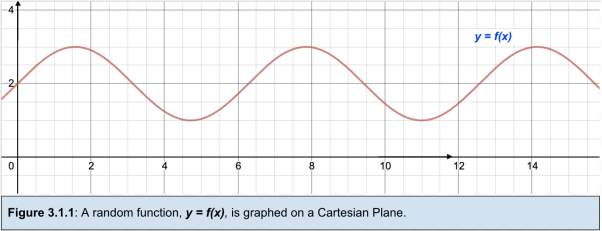Thin rectangles can be drawn underneath the curve from the x-axis to touching the curve.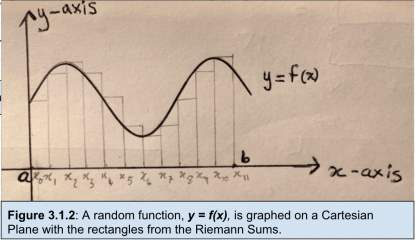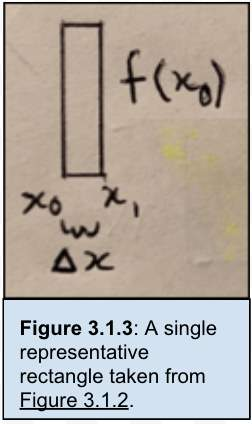These thin rectangles have an equal width of $∆x$

(difference between $x$

-values) and length $f\left(x\right)$

. This length can be calculated (above, right) by substituting the first $x$

-coordinate of every rectangle width into $y=f\left(x\right)$

as the rectangle touches the curve at this $x$

-coordinate.

However, by observation, these rectangles do not wholly account for the area under the curve as there are some gaps between the curve and rectangles, suggesting an underestimation and the rectangles are overshooting the curve, suggesting an overestimation of the area.

How can this area accurately be measured given these under/overestimations?

Rectangles of smaller widths can be created to minimise these over- and underestimations. This means that their widths ( $∆x$

) would be as small as possible, i.e. as the limit of $∆x$

approaches 0. Due to these very small widths, the number of rectangles would have to increase to make up the length of the area under the curve. Therefore, as the limit of $∆x$

approaches 0, the limit of the number of rectangles approaches $\infty$

. This aids in obtaining a more accurate value for the area and later on, volume and surface area, as the gaps between the curve and rectangles are minimised.

Therefore, in calculating the area under the curve, this technique of adding up an infinite number of rectangles using its area formula (length x width), is called the Riemann Sums.

This is seen in the following formula: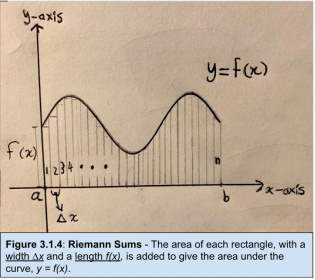Where:

The width is $∆x$

, the height is $f\left(x\right)$

. $x$

-coordinate with the lowest value in $f\left(x\right)$

place, taken from limits $a$

to $b$

.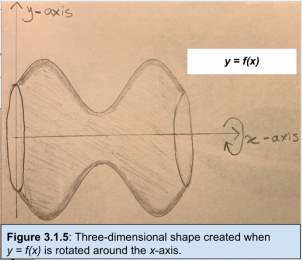This phenomenon can be used in the function y = f(x) to find its volume when rotated around the x-axis in the sketch on the left:

The volume of this three-dimensional object can be calculated by adding the volumes of an infinite number of small cylinders, which is similar to the rectangles in the Riemann Sums but extended in a three-dimensional sense.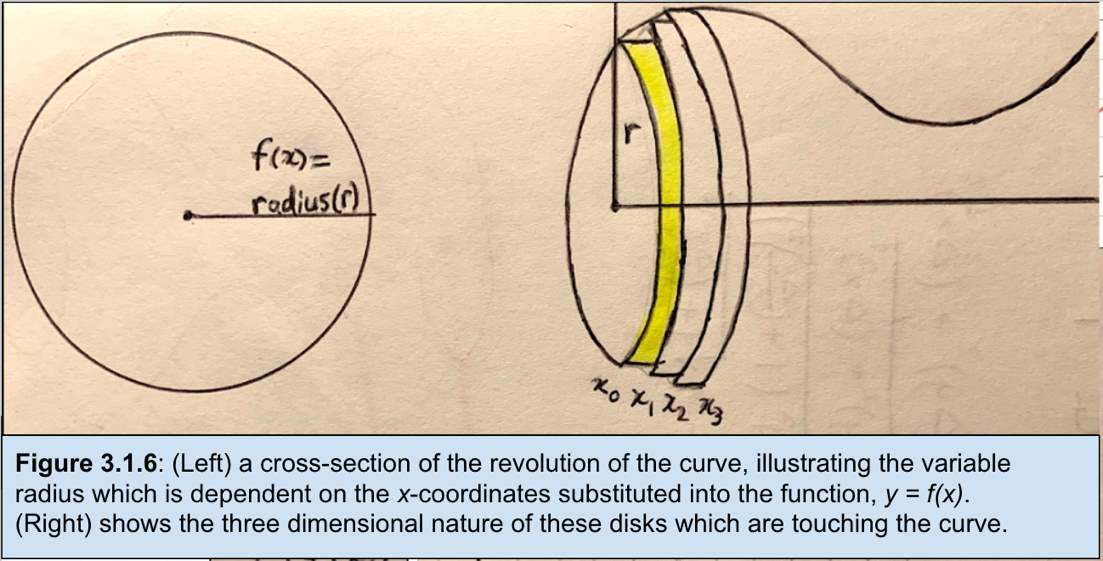Using the volume of a cylinder formula: V = $\pi {r}^{2}h$

and volume enclosed by lower and upper limits ‘a’ and ‘b’, the following volume formula is derived:

$\sum _{i=0}^{n–1}f{\left({x}_{i}\right)}^{2}×\pi ×∆x$

Where:

${r}$

= $f\left(x\right)$

as $x$

is the first $x$

-coordinate.

$h$

= $∆x$

(i.e. the difference between consecutive x values – e.g. ${x}_{1}–{x}_{0}$

).

$n–1$

= the number of cylinders, as there are $n$

cylinders

As $n–1$

approaches $\infty$

, this sigma notation is replaced by the integral sign and $∆x$

is replaced by an infinitesimally small width, $\mathit{dx}$

. This can be written in the formula:

### 3.2.Example Calculation:

Let $f\left(x\right)=\sqrt{{R}^{2}–{x}^{2}}$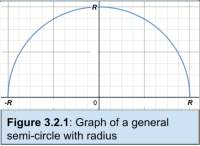The volume of the curve when rotated about the x-axis will be identical to a sphere. This can be proven through integrating from the previously derived volume formula:

$V=\pi \left[\left({R}^{3}–\frac{{R}^{3}}{3}\right)–\left(–{R}^{3}+\frac{{R}^{3}}{3}\right)\right]=$

Thus, it is evident that this is the general volume of a sphere formula.

### 3.3.Surface Area

The volume for finding the surface area of the three-dimensional object created from a 360˚ rotation about the x-axis is the following:

What does this mean? How is this equation derived?

In reference to the Riemann Sums, the similar basic principles are used to find the surface area of a function rotated about the x-axis (i.e. the surface of a revolution). This surface area is calculated through the addition of the outer, rounded surface area of an infinite number conical frustum (bottom section of cone).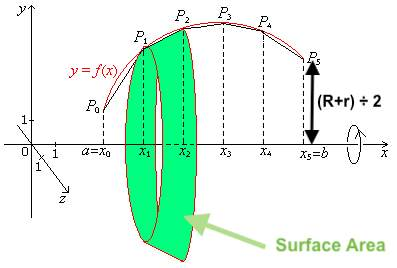{Image from: (Ving, 2015)} Calculating the surface area under a curve by dividing into frusta.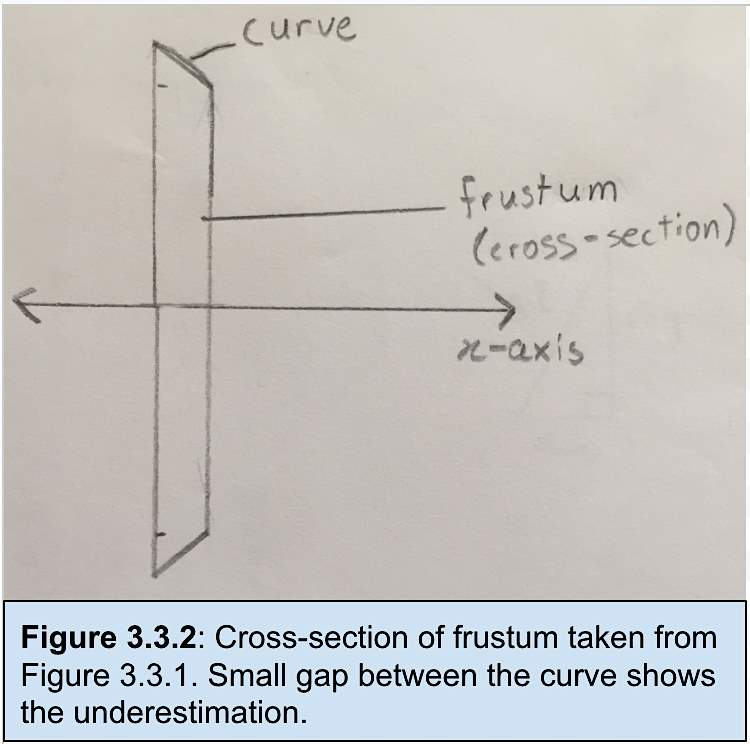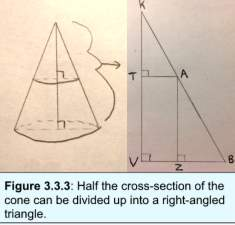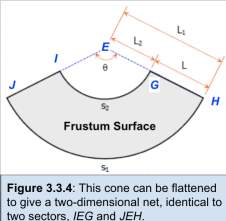{Images & Proof referenced from (Wilson J. , -)}

From the diagrams above, the surface area of the conical frustum can be derived.

Given that triangle A $\mid \mid \mid$

triangle B (equiangular)

$\frac{{L}_{1}}{R}=\frac{L}{R–r}$

${L}_{1}=\frac{\mathit{RL}}{R–r}$

 label this

${L}_{2}=\frac{\mathit{RL}}{R–r}–L$

 label this

$l=\mathit{r\theta }$

As the area of a sector is given by, $A=\frac{1}{2}{r}^{2}\theta$

$\mathit{Therefore},\mathit{Area of Major Sector}=\frac{1}{2}{\left({L}_{1}\right)}^{2}\mathit{\theta Label this}$

As ${s}_{1}={L}_{1}×\theta$

, we can rewrite this as equation:

$\mathit{Area Major Sector}=\frac{1}{2}{\left({L}_{1}\right)}^{2}\left(\frac{{s}_{1}}{{L}_{1}}\right)$

Similarly, $\mathit{Area Minor Sector}=\frac{1}{2}{\left({L}_{2}\right)}^{2}\left(\frac{{s}_{2}}{{L}_{2}}\right)$

Using the labelled equation above, we obtained the surface area of a frustum above:

${\mathit{SA}}_{\mathit{frustrum}}=\frac{1}{2}×\left({s}_{1}\right)×\left(\frac{\mathit{RL}}{R–r}\right)–\frac{1}{2}×\left({s}_{2}\right)×\left(\frac{\mathit{rL}}{R–r}\right)$

With reference to figure 3.3.3, we see that ${s}_{1}=2\mathit{\pi R}$

and ${s}_{2}=2\mathit{\pi r}$

, therefore this equation becomes:

This surface area formula can be written in sigma notation, much like Riemann Sums:

Where:

$f\left({x}_{i}\right)=r$

$f\left({x}_{i+1}\right)=R$

$∆{l}_{i}=\mathit{slant height}$

Through Pythagoras’ Theorem, $∆{l}_{i}$

can be written as $∆x×\sqrt{1+{\left(\frac{∆y}{∆x}\right)}^{2}}$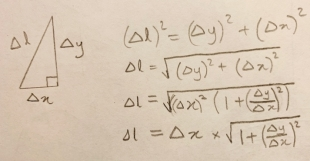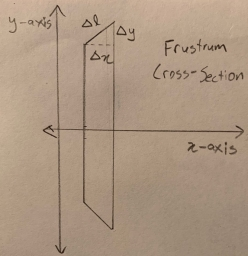In the limit as $n–1$

approaches ∞ we obtain the exact surface area of the revolution of this curve. In this limit, $∆x$

approaches 0 and $\frac{∆y}{∆x}$

approaches $\frac{\mathit{dy}}{\mathit{dx}}$

and $f\left({x}_{i+1}\right)$

approaches, $f\left({x}_{i}\right)$

, which is redefined as f(x).

Therefore, the final equation is for limits $a$

to $b$

:

### 3.4.Example Calculation

Let $f\left(x\right)=\sqrt{{R}^{2}–{x}^{2}}$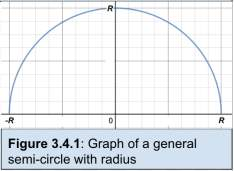The surface area of this function revolved around the $x$

-axis will result in the derivation of the surface area of sphere.

Using equation (3) with the upper and lower limits being $R$

and

respectively, $f\left(x\right)$

= $\sqrt{{R}^{2}–{x}^{2}}$

and $\frac{\mathit{dy}}{\mathit{dx}}=–\frac{x}{\sqrt{{R}^{2}–{x}^{2}}}$

, I calculated the surface area to be:

$\mathit{SA}=2\pi {\int }_{–R}^{R}\left(\sqrt{{R}^{2}–{x}^{2}}\right)×\sqrt{1+{\left(–\frac{x}{\sqrt{{R}^{2}–{x}^{2}}}\right)}^{2}}×\mathit{dx}$

$\mathit{SA}=2\pi {\int }_{–R}^{R}\left(\sqrt{{R}^{2}–{x}^{2}}\right)×\sqrt{1+\frac{{x}^{2}}{{R}^{2}–{x}^{2}}}×\mathit{dx}$

$\mathit{SA}=2\pi {\int }_{–R}^{R}\left(\sqrt{{R}^{2}–{x}^{2}}\right)×\sqrt{\frac{{R}^{2}}{{R}^{2}–{x}^{2}}}×\mathit{dx}$

$\mathit{SA}=2\pi {\int }_{–R}^{R}R×\mathit{dx}$

$\mathit{SA}=2\pi {\left[\mathit{Rx}\right]}_{–R}^{R}$

The above method shows that the familiar formula of the surface area of a sphere can be obtained.

## 4.Modelling the Shape of The Vase

### 4.1.Obtaining measurements

In order to effectively explain the measurements, I obtained using a 30-centimetre ruler, tape measure, a piece of non-stretch string, a marker and a camera phone as my measuring equipment.

Below, I have sketched the cross-section of the vase and labelled it with measurements.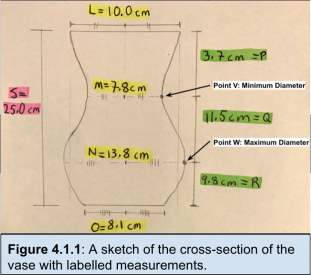L = 10.0 centimetres, O = 8.1 centimetres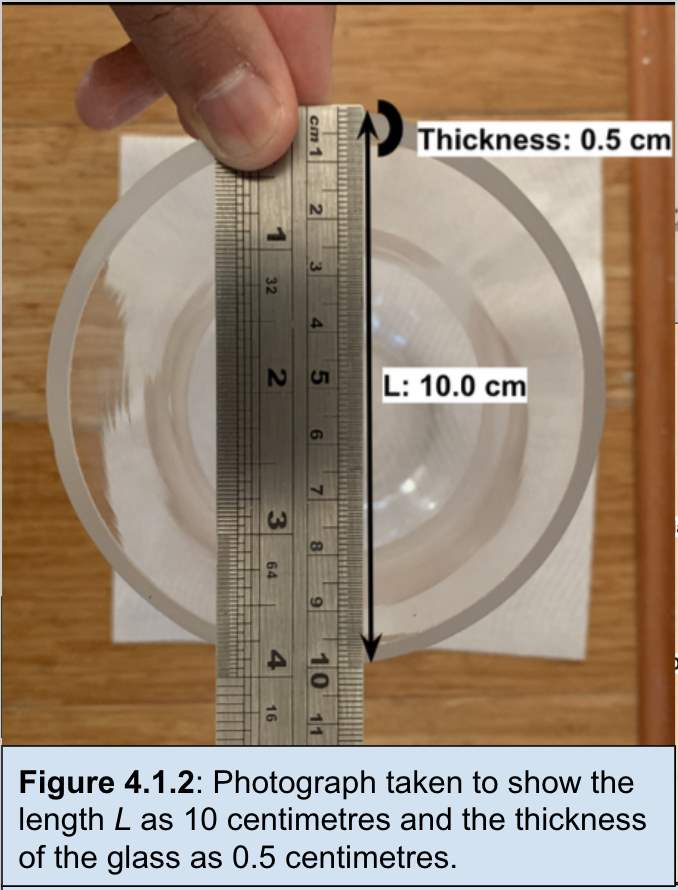I was able to measure the diameter of the open top section to one decimal place. In doing so, I have maintained consistency and a degree of accuracy. Knowing that the open top is a circle, I found the radius to be 5 cm. The same method was applied to find length O at the base of the vase. In finding the thickness of the glass, there is a distinction being made between the volume of the vase and the volume contained by the vase. This will be important when testing the validity of my results for the vase’s volume. M = 7.8 centimetres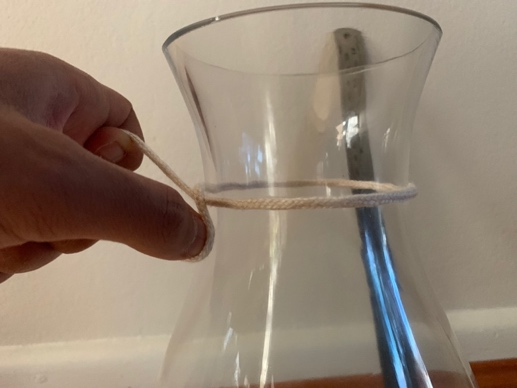In order to accurately model the shape of the vase, I had to find the point on the vase that had the smallest width. I tied a piece of non-stretch string tightly around the vase, at the minimum width and marked a point on both ends of the string as well as the vase to give the location of the point for later measurements. This measurement only gives the circumference of the outside of the vase. This means the diameter (M) of the circle is 7.8 cm. In measuring this circumference, length M, I have made the assumption that this vase, is composed of a circular disks of varying diameters.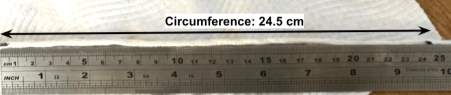N = 13.8 centimetres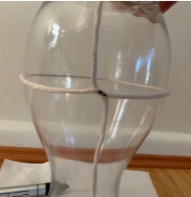Similarly, in measuring the largest width, I had to use observation to locate this point of maximum width, tie the non-stretch string around it, mark the point at which both ends of the string touch and the location of this maximum point. Using the same method, the length N is found to be 13.8 cm by dividing circumference, 43.5 cm by π.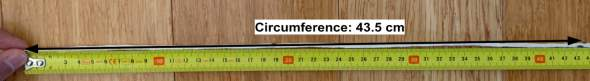Q = 11.5 centimetres, P = 3.7 cm, R = 9.8 cm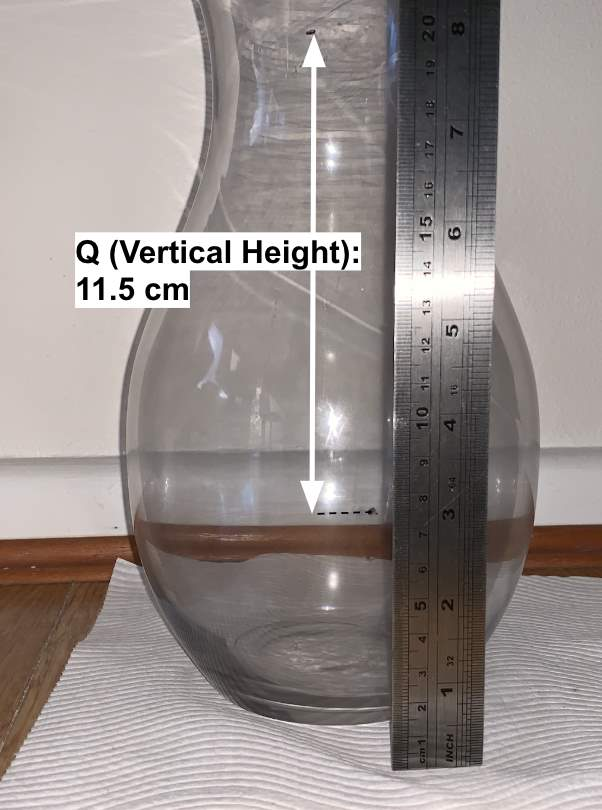In finding the minimum and maximum widths of the vase using the non-stretch string, I marked the points at which these occur and used observation from taking a photo to find the vertical height (Q). Length P was also found using this method. Length R was found by summing lengths P and Q and subtracting it by the overall height to obtain R.

One major assumption that I made was in determining where my point of minimum width was. Given the available equipment, I used observation to determine the points of minimum and maximum widths, which is a quicker and more effective way of determining these points. Although, in reality, one can use technology such as photography scaling, to locate these points to greater precision. No matter where this point is situated, the measurements would be in the same approximate range of values (i.e. 24-25 centimetres). Given the small measurements involved in these calculations of area and volume, a difference of one centimetre would not drastically change these area and volume values.

Our academic experts are ready and waiting to assist with any writing project you may have. From simple essay plans, through to full dissertations, you can guarantee we have a service perfectly matched to your needs.

Another assumption was made in determining length Q. Through observation and photography, I was able to find this length. Concerning accuracy, I made sure I held the ruler as straight as possible (perpendicular to the floor) in one hand and the camera in the other hand and attempted to take a photo at level with the ruler to avoid any parallax error. Given the difficulty of this method, there is a greater margin of error in Q’s length and is incurred into the equation, volume and surface area.

### 4.2.Finding an equation

A trigonometric equation must be derived from these measurements in order to find the surface area and volume. Through experimentation, I found the cosine curve, the most accurate.

Using the general equation:

A, B and D values were derived.

Given that A is the amplitude: half of length Q (radius) – half of length M (radius), i.e. 6.9 – 3.9 = 3, to give the vertical distance between the two points and halved to obtain the distance of 1.5 cm.

B was found from doubling length Q to obtain a period of 23 cm. Therefore, B = $\frac{\mathbit{2}\mathbit{\pi }}{\mathbit{23}}$

.

D was found through adding 6.9 and 3.9 and dividing by 2 to find a vertical translation of 5.4 cm.

Through simultaneous equations, the missing C value can be calculated.

From my measurements, one point from points: (0,5) from the top of vase of length L or (25,6.9) from the base and sides of lengths N and S respectively, can be used.

In substituting point (0,5):

$\therefore C=–4.13893405$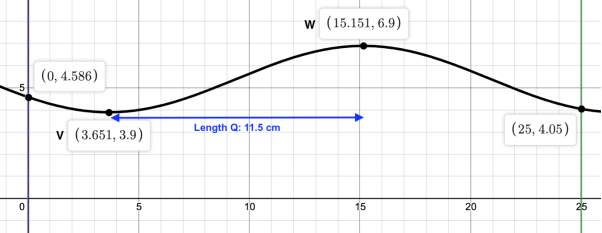This function’s graph represents a limitation of the modelling process due to the finite number of parameters (limits) available in the general form: A, B, C and D. Each parameter equates to one point. Given that the maximum and minimum points (W and V) are two parameters, the length from these points (length Q) measured at 11.5 cm is another parameter. This means that either the point of the base (25, 4.05) or the top point (0,5) must be graphed to find ‘C’. In graphing one of the above, it does not necessarily mean that the other point will lie on the curve, which is exactly the case above as the cosine curve has a y-intercept of (0, 4.586) instead of the desired (0,5) obtained from measurement. As a result, a slight underestimation of the volume and surface area occurs (refer to Reflection).

## 5.Find volume and surface area using formulae derived

### 5.1.Volume

Calculated through Wolfram Alpha Online Integration Calculator.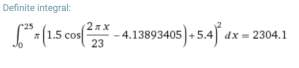### 5.2.Surface Area

$\mathit{SA}=2\pi {\int }_{a}^{b}f\left(x\right)×\sqrt{1+{\left(\frac{\mathit{dy}}{\mathit{dx}}\right)}^{2}}×\mathit{dx}$

$\mathit{SA}=2\pi {\int }_{0}^{25}\left(A\mathrm{cos}\left(\mathit{Bx}–C\right)+D\right)×\sqrt{1+{\left(\frac{d}{\mathit{dx}}\left(A\mathrm{cos}\left(\mathit{Bx}–C\right)+D\right)\right)}^{2}\mathit{dx}}$

Use of Wolfram Alpha to differentiate f(x).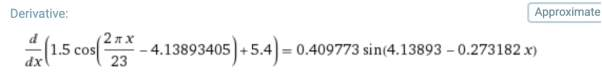(2 decmial places)

Rounding as ruler can only measure up to 0.1 of 1 centimetre.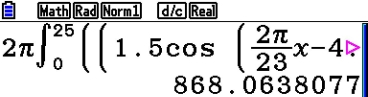Use of Graphic Display Calculator.

Total Surface Area = Circle base + Curved surface ≈ 919.5 cm2

Another limitation in determining the values of volume and surface area was my level of maths. Given the complex nature of the integrational calculus above, the use of technology in finding a straightforward answer by entering the equation into a Graphic Display Calculator (GDC) or Wolfram Alpha online calculator, was imperative in yielding a result. In using the exact value or full number correct to the maximum number of decimal places was essential in yielding an accurate result but could lead to certain inaccuracies caused by simple mistakes in entering the formula into the GDC.

## 6.Discussion of Results

### 6.1.Finding a distinction between capacity and volume

Looking at the results I have obtained from this Exploration, I wanted to test the accuracy of the results for the volume of the vase. I would achieve this by finding the initial weight of the empty vase and completely fill it with water and weigh it. In doing so I would obtain the volume able to be contained inside the vase, given that the density of water is 1 gram per cm3, meaning that the weight and volume absolute values are equal.

 Finding the capacity of the vase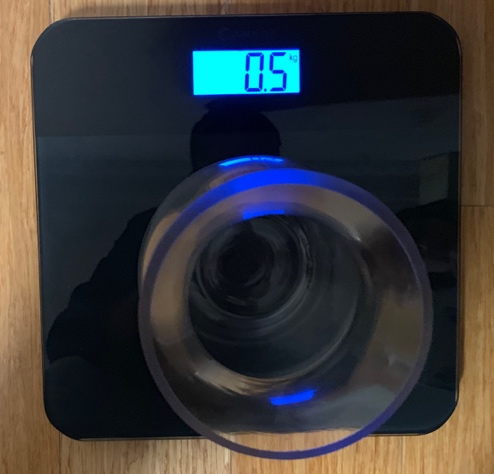0.5 kg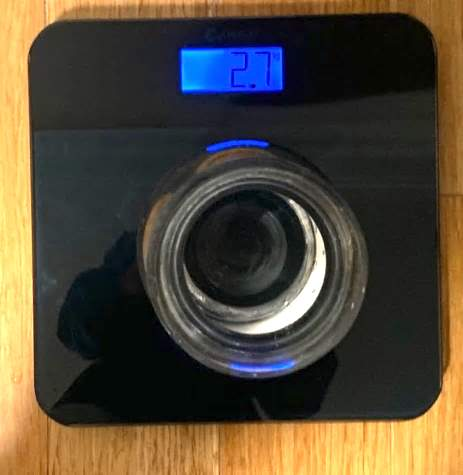2.7 kg The capacity of the vase is 2,200 cm3 which is approximately 104.1 cm3 lower than the answer obtained for the vase volume. Why is there such a large difference? The overall thickness of the glass contributes to the overall volume of the vase adding 1 centimetre to the diameters of the circular disks that make up the vase. This is accounted for when finding the volume and was the major factor in creating this difference between volume and capacity.

In order to keep this method consistent, there needs to be a way to find the surface area of the vase where there is a greater degree of accuracy. The reliability of my results decreases as there are no other methods to test their accuracy, given the equipment at hand.

### 6.2.Re-modelling the vase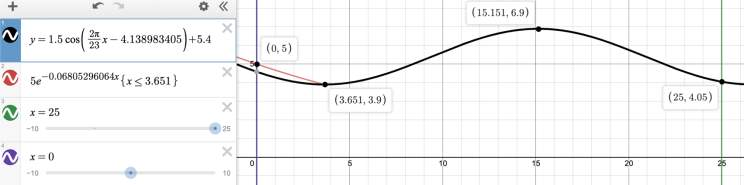Another crucial limitation in my experiment was the modelling of the vase. As discussed in section 4.2, there are only 4 available parameters and 5 available points to fit those parameters meaning that the final equation of the function will not pass through all 5 points. In order to rectify this, I proposed that another function, for simplicity an exponential, be created which passes through the point of minimum width (3.651, 3.9) and through the point (0, 5) as follows:

In doing so, all five parameters are met and a more accurate value for the volume and surface area is attained. In finding the equation of the exponential by transforming general function:

This curve should pass through the points (0,5) and (3.651, 3.9). Thus, substituting the point (0,5), I obtained $b$

= 5.

The function is reflected about the $y$

-axis then, multiplied by 5 to obtain a $y$

-intercept of   (0, 5).

The unknown $a$

value must be obtained by substituting the minimum width point and solving for $a$

.

$\because 3.9={e}^{–a\left(3.651\right)}$

$a=–\frac{\mathrm{ln}\left(3.9\right)}{3.651}$

$\therefore a=0.06805296064$

The use of a combination of these two functions combines the integral equations into the following volume equation: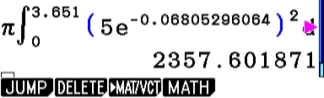In general, my yielded results are very valid, and that the correct methods were applied to reach these calculations. One option to limit the underestimations would be to measure more points on the vase to give a more accurate indicator. However, given the inherent nature of error in measurement and mathematical theories, the more measurements and calculations that are made, the greater is the margin for error. Thus, the following has limited my aim in acquiring the true value. It has also placed doubt as to whether any type or form of measurement can be 100% justified.

## 7.Evaluation & Link to Real-World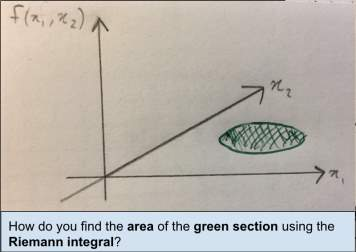Throughout my investigation, I have made the attempt to learn how integral calculus has been derived and applied to finding the volume and surface area of any function that is rotated around the $x$

-axis. Not only have I learnt about these techniques, I have also learnt about the history and logic behind the derivation of a fundamental part of the mathematical world. These formulae are derived from the basic “Riemann Sums” principle originally discovered by German mathematician Bernhard Riemann in 1854, where the sums of the rectangles under the curve are added up to obtain an area (Downey, 2019). The Riemann Integral was then derived.

As found by my observations in its application in the real-world, the Riemann Integral is limited in its scope. The inability to integrate non-continuous functions (e.g. some piecewise functions contain points of discontinuity) affects the ability to expand into higher domains where all $x$

-values can be bound in the shape of say a circle or ellipse (The Bright Side Of Mathematics, 2018). This led to the derivation of other integrals such as the Lebesgue Integral, which appear to contain less limitations.

It is also worthy to note, that Riemann was also known for his developments in “Riemannian Geometry”, a form of non-Euclidean geometry used to describe a curved surface, which served as a basis for Einstein’s “Theory of Relativity” (Mastin, 2010); (Setia, 2008).

On a global note, a possible extension of this Exploration could be in the area of the use/misuse of glass in the production of three-dimensional objects such as vases, drinking glasses and artworks and how they can have a large impact on the levels of pollution in the world. The combustion of natural gas and the decomposition of raw materials leads to the emission of CO2 (AGC, 2019). In some cases, the nitrogen oxides and sulfur dioxide emitted from the decomposition process cause acidification (decrease in the pH of the world’s oceans – more acidic, more aquatic life dead) and increased formation of smog (AGC, 2019). Given that glass is 100% recyclable, the recycling of glass is predicted to reduces related air and water pollution by 20% and 50% respectively. (WWF, 2019). My exploration allowed me to understand how much glass goes into making a standard vase and consider the effects of making each one, on the environment. From my findings, I found that approximately, 0.5 kg of glass (weighed in section 6.1) making up 0.004% of the 11,470 tonnes of glass in US landfills according to 2015 data (EPA, 2015).

Suppose, if the vase is made into a right cylinder, with a diameter of 8.1 cm (length O) and vertical height 25 cm, the surface area of this rounded surface would be 25.4 cm2 instead of 868.1 cm2. This highlights the 97.1% increase in surface area for the sake of aesthetic appeal from the curved nature of the vase. This cost is then incurred to the environment where there is an inefficiency in glass usage and hence, pollution.

## 8.Conclusion

Overall, given the process undertaken in my Mathematical Exploration, I have gained knowledge and insight into integration and its derivation and application in the real-world. I have gained a better grasp on the basic, founding principles of integration, its inherent inaccuracies and have used it to explore and expand my understanding of the world in which I live in the present, as well as the mathematics and mathematicians in the past who moulded this reality.

## References & Bibliography:

• AGC. (2019, – -). Our environmental impact. Retrieved from AGC Glass Europe: http://www.agc-glass.eu/en/sustainability/environmental-achievements/environmental-impact
• Davis, L. (2017, August 9). Australia’s glass recycling crisis: the industry responds. Retrieved from Sustainability Matters: https://www.sustainabilitymatters.net.au/content/waste/article/australia-s-glass-recycling-crisis-the-industry-responds-809131767
• Downey, T. S. (2019, – -). Riemann Sums. Retrieved from Math Open Reference: https://www.mathopenref.com/calcriemann.html
• Mastin, L. (2010, – -). 19th Century Mathematicians – Riemann. Retrieved from The Story of Mathematics: https://www.storyofmathematics.com/19th_riemann.html
• Pubmed.gov. (2014, February 21). Retrieved from Pollution due to hazardous glass waste.: https://www.ncbi.nlm.nih.gov/pubmed/24281678
• Setia, V. (2008, July 13). Riemann Geometry. Retrieved from Encyclopædia Britannica: https://www.britannica.com/science/Riemannian-geometry
• Ving, P. K. (2015, May 14). Calculus of One Real Variable. Retrieved from Areas Of Surfaces Of Revolution: http://www.phengkimving.com/calc_of_one_real_var/12_app_of_the_intgrl/12_07_areas_of_surf_of_revltn.htm
• Wilson, J. (-, – -). Conical Frustum. Retrieved from University of Georgia: http://jwilson.coe.uga.edu/EMAT6680Fa2013/Kar/EMAT%206690/Essay%202/Frustum.pdf
• WWF. (2019, – -). Recycling Glass – How it helps the environment. Retrieved from WWF: http://wwf.panda.org/knowledge_hub/teacher_resources/project_ideas/recycling_glass.cfm

View all

## DMCA / Removal Request

If you are the original writer of this essay and no longer wish to have your work published on UKEssays.com then please:

Related Services

Prices from

S\$203

Approximate costs for:

• 1000 words
• 7 day deliveryHumanity University

Dedicated to your worth and value as a human being!

Related Lectures